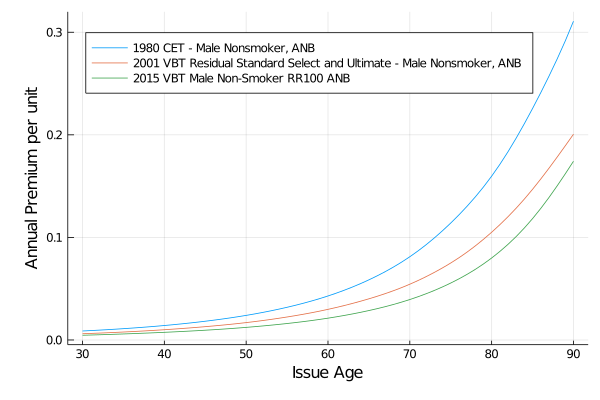## ActuarialScience.jl

Life Actuarial Maths
Author JuliaActuary
Popularity
12 Stars
Updated Last
5 Months Ago
Started In
November 2016

# LifeContingencies.jl

LifeContingencies is a package enabling actuarial life contingent calculations. The benefits are:

• Integration with other JuliaActuary packages such as MortalityTables.jl
• Fast calculations, with some parts utilizing parallel processing power automatically
• Use functions that look more like the math you are used to (e.g. `Ax`, `ä`) with Unicode support
• All of the power, speed, convenience, tooling, and ecosystem of Julia
• Flexible and modular modeling approach

## Package Overview

• Leverages MortalityTables.jl for the mortality calculations
• Contains common insurance calculations such as:
• `A(x)`: Whole life
• `A(x,n)`: Term life for `n` years
• `ä(x)`: Life contingent annuity due
• `ä(x,n)`: Life contingent annuity due for `n` years
• Contains various commutaion functions such as `D(x)`,`M(x)`,`C(x)`, etc.
• Various interest rate mechanics (e.g. stochastic, constant, etc.)
• More documentation available by clicking the DOCS bages at the top of this README

## Examples

Calculate the whole life insurance rate for a 30-year-old male nonsmoker using 2015 VBT base table and a 5% interest rate

```using LifeContingencies, MortalityTables

tbls = MortalityTables.tables()
vbt2001 = tbls["2001 VBT Residual Standard Select and Ultimate - Male Nonsmoker, ANB"]
issue_age = 30
l = LifeContingency(
vbt2001.select,
InterestRate(0.05),
issue_age
)

start_time = 0
A(l,start_time) # 0.111...```

Use a stochastic interest rate calculation to price a term policy:

```using LifeContingencies, MortalityTables
using Distributions

tbls = MortalityTables.tables()
vbt2001 = tbls["2001 VBT Residual Standard Select and Ultimate - Male Nonsmoker, ANB"]

# use an interest rate that's normally distirbuted
μ = 0.05
σ = 0.01
int = InterestRate(t -> rand(Normal(μ,σ)))

l = LifeContingency(
vbt2001.select,
int,
30 # issue age
)

start_time = 0
term = 10
A(l,start_time,term) # somewhere around 0.055```

You can use autocorrelated interest rates - substitute the following in the prior example using the ability to self reference:

```σ = 0.01
initial_rate = 0.05
int = InterestRate(
function intAR(time)
if time <= 1
initial_rate
else
i′ = last(int.rate_vector)
rand(Normal(i′,σ))
end
end
)
```

Compare the cost of annual premium, whole life insurance between multiple tables visually:

```using LifeContingencies, MortalityTables, Plots

tbls = MortalityTables.tables()
tables = [
tbls["1980 CET - Male Nonsmoker, ANB"],
tbls["2001 VBT Residual Standard Select and Ultimate - Male Nonsmoker, ANB"],
tbls["2015 VBT Male Non-Smoker RR100 ANB"],
]

issue_ages = 30:90
int = InterestRate(0.05)

whole_life_costs = map(tables) do t
map(issue_ages) do ia
lc = LifeContingency(
t.ultimate,
int,
ia
)

A(lc,0) / ä(lc,0)

end
end

plt = plot(ylabel="Annual Premium per unit", xlabel="Issue Age",
legend=:topleft, legendfontsize=8)
for (i,t) in enumerate(tables)
plot!(plt,issue_ages,whole_life_costs[i], label="\$(t.d.name)")
end
display(plt)```## References

### Used By Packages

No packages found.